The gradient is a Vector operator denotedand sometimes also called Del or Nabla. It is most often applied to a real function of three variables, and may be denoted(1)

For general Curvilinear Coordinates, the gradient is given by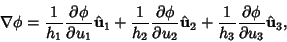(2)

which simplifies to(3)

in Cartesian Coordinates.

The direction of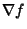is the orientation in which the Directional Derivative has the largest value andis the value of that Directional Derivative. Furthermore, if, then the gradient is Perpendicular to the Level Curve through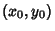ifand Perpendicular to the level surface through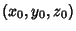if.

In Tensor notation, let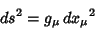(4)

be the Line Element in principal form. Then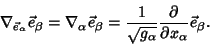(5)

For a Matrix,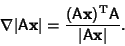(6)

For expressions giving the gradient in particular coordinate systems, see Curvilinear Coordinates.

Arfken, G. Gradient,'' and Successive Applications of.'' §1.6 and 1.9 in Mathematical Methods for Physicists, 3rd ed. Orlando, FL: Academic Press, pp. 33-37 and 47-51, 1985.